二维数组的初始化

1)按行进行初始化

2)连续赋值初始化

3)部分元素初始化

4)全员赋0初始化

5)第一维不定义初始化

数组名

二维字符数组

二维字符数组的定义

二维字符数组的初始化

# 数组概述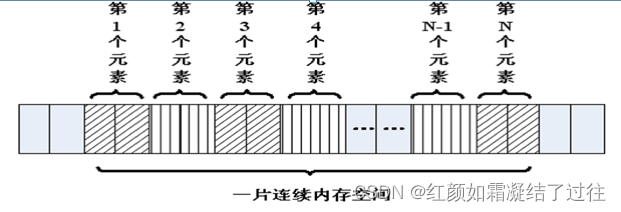--我们可以将一个数组分解为多个数组元素：这些数组元素可以是基本数据类型或构造类型。

int a;  //基本数据类型
struct Stu p;    //构造


（注：构造数据类型：数组类型、枚举类型enum、指针类型、引用类型、结构体struct、联合体union（又称联合，共用体）、class类等。）

--按数组元素类型的不同，数组可分为：数值数组、字符数组、指针数组、结构数组等类别。

int a ;    //数值数组
char s ;    //字符数组
char *p ;    //指针数组
struct Peo n ;    //结构数组


# 一维数组

## 如何使用一维数组

1. 数组名字符合标识符的书写规定(数字、英文字母、下划线) 。
2. 数组名不能与其它变量名相同 。
3. 方括号[]中常量表达式表示数组元素的个数 。
//方括号中的数量是其数组所包含元素，但其下标应该从0开始 。

a 包含 a, a, a 三个元素

//0， 1， 2分别表示其元素下标 。

#include <stdio.h>

int main()
{
int a ;    //在这里我们定义了一个数组a，a有100个成员，每个成员都是int类型
//没有a这个变量，a是数组的名字，但不是变量名，它是常量

int i = 0;
for (i = 0; i < 100; i++)
{
a[i] = i; //给数组赋值 让a = 0, a = 1 ... a[i] = i ;
}

//遍历数组，输出每个成员的值
for (i = 0; i < 100; i++)
{
printf("%d ", a[i]);
printf("\n");
}
printf("\n");

return 0;
}


## 一维数组的初始化

#include <stdio.h>

int b ;	//定义全局数组

int main()
{
int a = { 1, 2, 3, 4, 5 }	;	//定义一个数组并进行初始化
int c ;	//定义局部数组
int d[]	= {1, 2, 3}	;	//如果[]中不定义元素个数，定义时必须初始化
int e = { 0 };//所有的成员都设置为0

printf("%d\n", a) ;
printf("%d\n", b) ;
printf("%d\n", b) ;
printf("%d\n", c) ;
printf("%d\n", c) ;

return 0;
}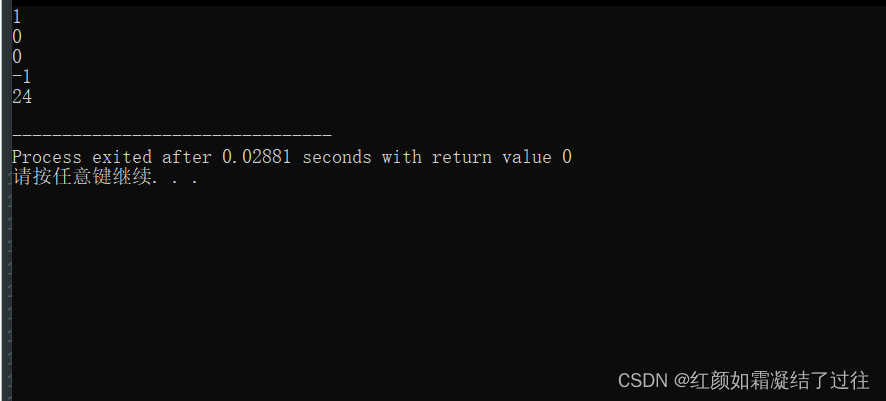这个运行结果就很好的展示了我们上面所讲到的知识。

## 数组名

sizeof 是一个关键字，它是一个编译时运算符，用于判断变量或数据类型的字节大小。sizeof 运算符可用于获取类、结构、共用体和其他用户自定义数据类型的大小。

sizeof的语法：

sizeof (object) ;

#include <stdio.h>

int main()
{
int a = { 1, 2, 3, 4, 5 };	//定义一个数组并初始化所有成员变量

printf("%p\n", a) ;		//数组名是一个地址的常量，代表数组中首元素的地址。
printf("%p\n", &a) ;	// a和a的地址是相同的

int n = sizeof(a) ; 		//数组占用内存的大小，5个int类型，5 * 4  = 20
int n0 = sizeof(a) ;		//数组第0个元素占用内存大小也就是这个数组中每个元素内存的大小。

int i = 0;
for (i = 0; i < sizeof(a) / sizeof(a); i++)	// sizeof(a) / sizeof(a就是数组中元素个数
{
printf("%d ", a[i]);
}
printf("\n");

return 0;
}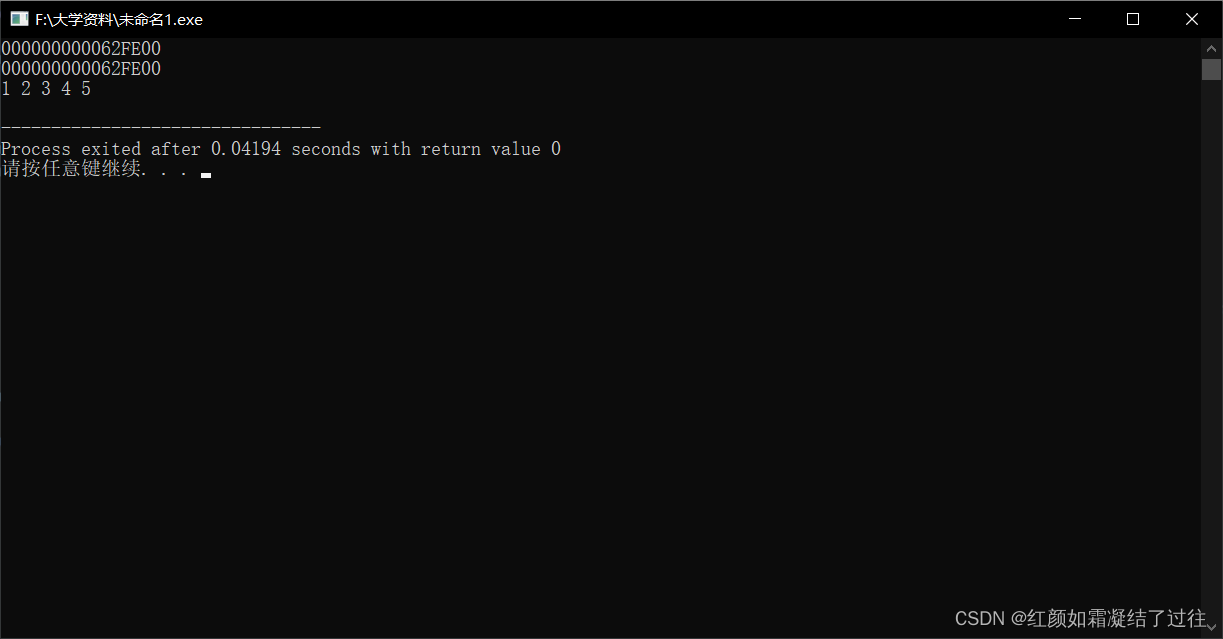## 一维字符教组

### 一维字符教组的定义

char 数组名[数组大小] ;

char a ;

### 一维字符数组初始化

1）逐个字符赋值

a.当字符个数少于数组空间大小时

char a = {'h','e','l','l','o'} ;    //始值个数5小于数组a空间个数8

printf ("%s”，a) ;

char a[] = {'h','e','l','l','o'} ;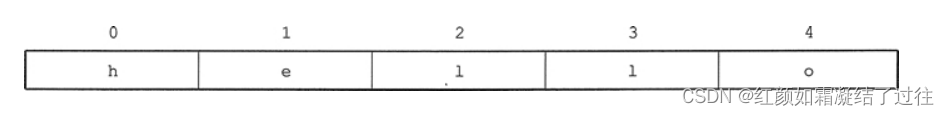b.当字符个数等于数组空间大小时

char a={'h','e','l','l','o'} ;    //初值个数5等于数组大小5

此语句的执行结果如下图：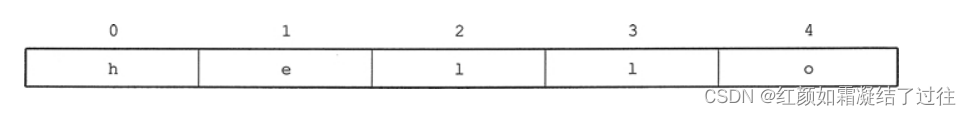可以发现，这时char a={'h','e','l','l','o'} 与 char a[] = {'h','e','l','l','o'}就成为了等价关系。同样该字符数组中不包含字符串结束标志 '\0'，故不能使用 printf("%s"，c); 输出其中的字符串。

int i;
for(i=0;i<5;i++)
{
printf ("%c",a[i]) ;
}



c.当字符个数多于空间大小时

char a = {'h','e','l','l','o'}; //初值个数5大于数组大小4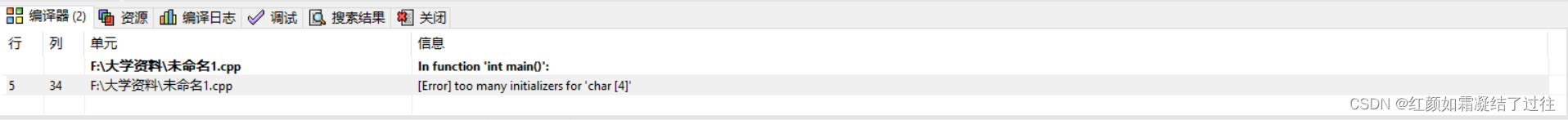2）字符串初始化赋值

char a = {"Hello World"}; //注意该数组大小应足够大 不然会报错

char a = "Hello World"  ;

char a = {"Hello World"} ;
//等价于
char a = "Hello World"  ;
//等价于
char a= {'H','e','l','l','o',' ','W','o','e','l','d','\0','\0','\0','\0'} ;
//等价于
char a= {'H','e','l','l','o',' ','W','o','e','l','d','\0'} ;
//等价于
char a= {'H','e','l','l','o',' ','W','o','e','l','d'} ;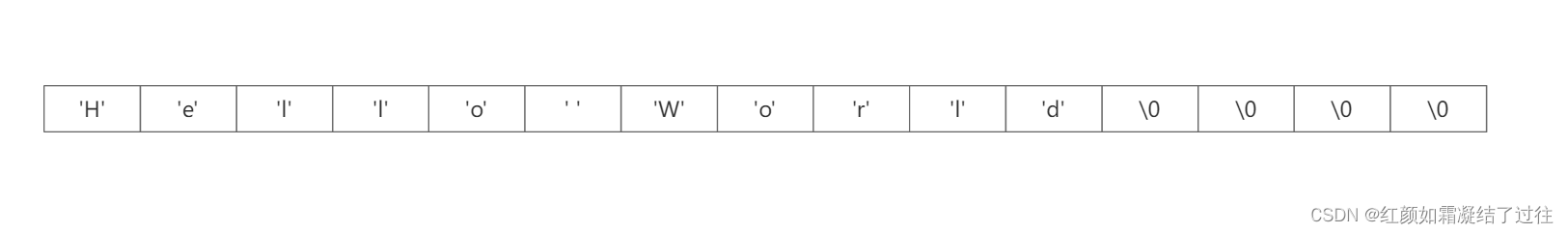为了防止字符个数超出数组空间的现象，我们在采用字符串对字符数组进行初始化时，一般省略一维数组空间的大小，即:

char a[] = {"Hello World"} ;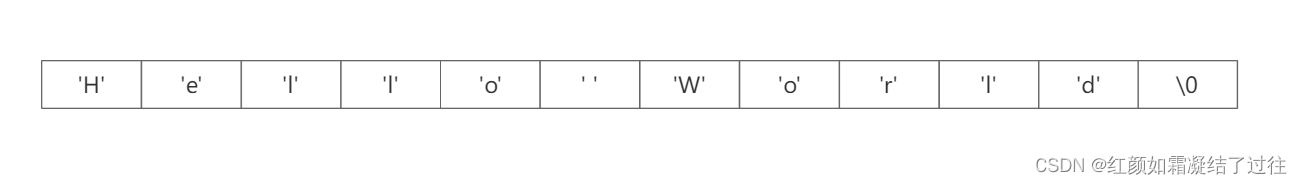为节省空间以及书写方便防止不必要的失误，当我们采用用字符串对字符数组初始化时，一般均省略其一维的大小。

### 一维字符数组的引用

char a[]="abcd" ;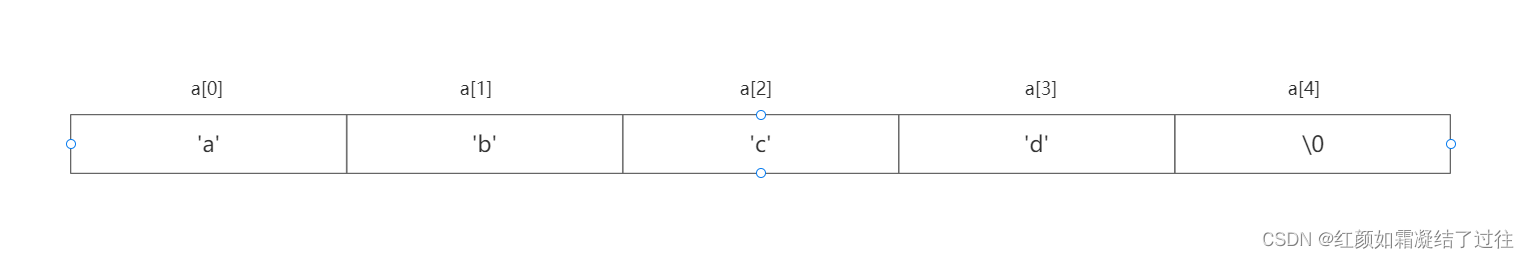int i;
for(i=0;c[i]!='\0';i++) //当前i号位置的字符变量只要不是结束符就输出
printf("%c",a[i]) ;

其中for循环使用 '\0' 作为判断依据也可以很好的避免了计算长度所带来的复杂程度。

# 二维数组

## 如何使用二维数组

类型说明符 数组名[常量表达式1][常量表达式2]

int a ;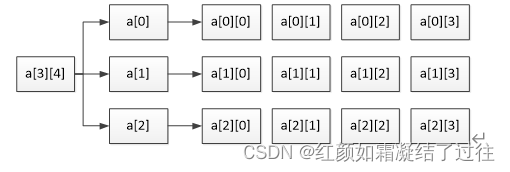与一维数组相同，二维数组的行序号和列序号的下标都是从 0 开始的。元素 a[i][j] 表示第 i+1 行、第 j+1 列的元素。数组 int a[m][n] 最大范围处的元素是 a[m–1][n–1]。所以我们在日常引用数组元素时应该注意，下标值应在定义的数组大小的范围内。

#include <stdio.h>

int main()
{
int a;	//定义二维数组

/*
这个二维数组由三个一维数组组成
这三个一维数组的数组名分别是 a,a,a
而这个一维数组是 int 
*/

int i = 0;
int j = 0;
int num = 0;

for (i = 0; i < 3; i++)		//for循环给数组每个元素赋值
{
for (j = 0; j < 4; j++)
{
a[i][j] = num++;
}
}

for (i = 0; i < 3; i++)
{
for (j = 0; j < 4; j++)
{
printf("%d	 ", a[i][j]) ;	//输出每个成员的值
}
printf("\n");
}

return 0;
}

运行结果：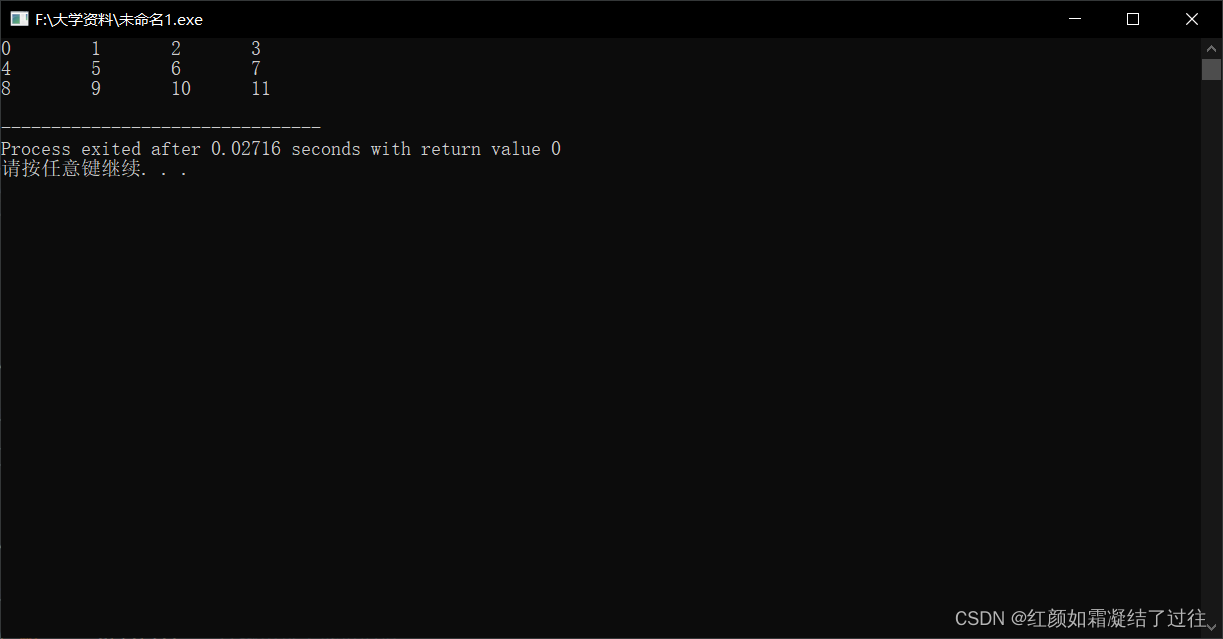## 二维数组的初始化

数据类型 数组名[常量表达式1][常量表达式2] = { 初始化数据 } ;

### 1)按行进行初始化

int a = {{1, 2, 3, 4}, {5, 6, 7, 8}, {9, 10, 11, 12}};

### 2)连续赋值初始化

int a = {1, 2, 3, 4, 5, 6, 7, 8, 9, 10, 11, 12};

### 3)部分元素初始化

int a = { 1, 2, 3, 4  } ;
int a = {{1, 2}, {5}, {9}};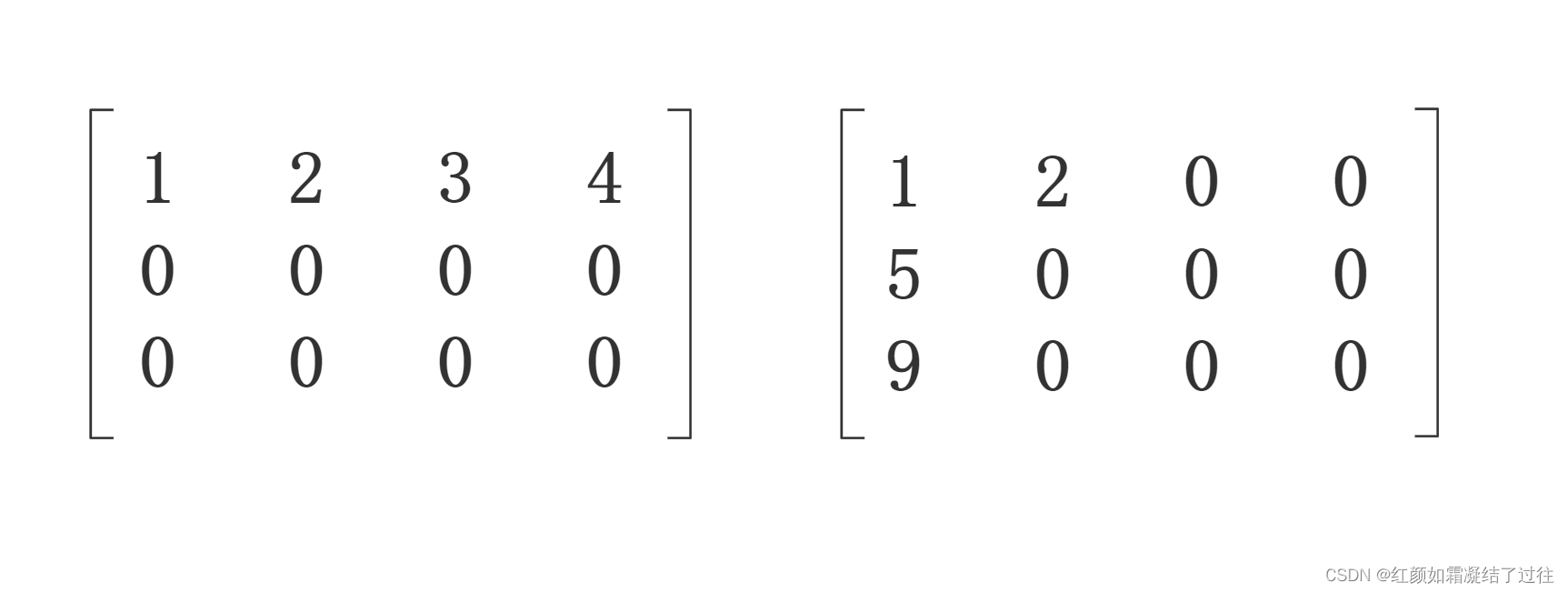### 4)全员赋0初始化

int a={0}；

### 5)第一维不定义初始化

int a[] = {1, 2, 3, 4, 5, 6, 7, 8, 9, 10, 11, 12};

    //按行进行初始化
int a = {{ 1, 2, 3, 4 },{ 5, 6, 7, 8, },{ 9, 10, 11, 12 }} ;

//连续赋值初始化
int a = { 1, 2, 3, 4 , 5, 6, 7, 8, 9, 10, 11, 12  } ;

//部分元素初始化
int a = { 1, 2, 3, 4  } ;

//全员赋0初始化
int a = {0} ;

//第一维不定义初始化
int a[] = {1, 2, 3, 4, 5, 6, 7, 8, 9, 10, 11, 12};


## 数组名

#include <stdio.h>

int main()
{
//定义了一个二维数组
int a = { 1, 2, 3, 4 , 5, 6, 7, 8, 9, 10, 11, 12  } ;

//数组名为数组首元素地址，二维数组的第0个元素为一维数组
printf("a = %p\n", a) ;    //输出地址
printf("a = %p\n", a) ;

//测二维数组所占内存空间
//sizeof(a) = 3 * 4 * 4 = 48
printf("sizeof(a) = %d\n", sizeof(a)) ;

//测第0个元素所占内存空间
printf("sizeof(a) = %d\n", sizeof(a) ) ;

//测第0行0列元素所占内存空间
printf("sizeof(a) = %d\n", sizeof(a)) ;

return 0;
}


运行结果：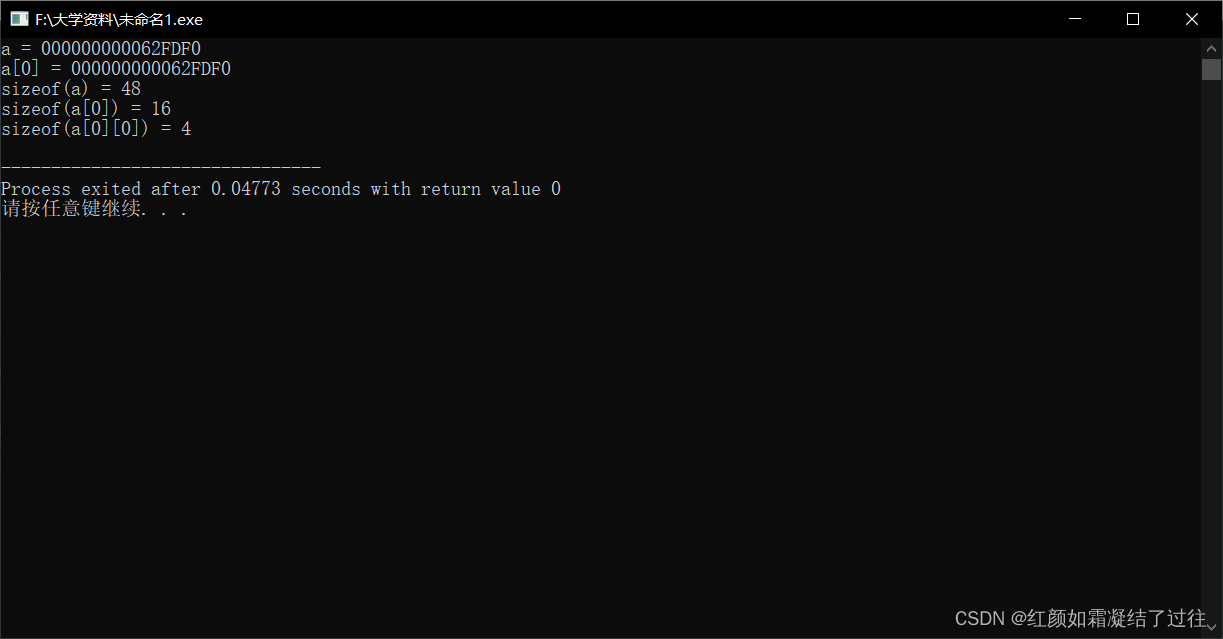//求二维数组行数
printf("i = %d\n", sizeof(a) / sizeof(a)) ;

// 求二维数组列数
printf("j = %d\n", sizeof(a) / sizeof(a)) ;

//求二维数组行*列总数
printf("n = %d\n", sizeof(a) / sizeof(a)) ;


## 二维字符数组

### 二维字符数组的定义

char 数组名[第一维大小][第二维大小];

char a ;

### 二维字符数组的初始化

char a = {"Hello","C++" } ;
char a[] = {"Hello","C++"} ;//第二维同样不可省略

//等价于
char a = {{"Hello"},{"C++"} } ;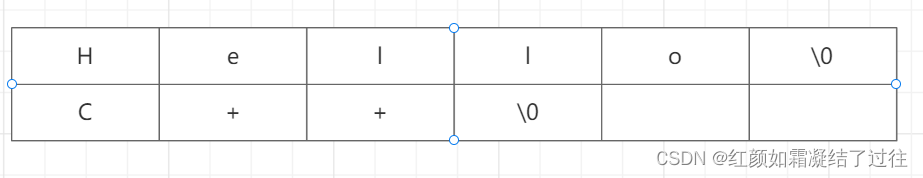a由于在二维字符数组每个字符串单独占一行, 所以可以用 a[n] 引用该二维数组字符中第 n 行的字符串；也可以用 a[i][j] 引用某行某列中的单独一个字符串 。

### 二维字符教组的引用

printf ("%c",a) ;     //输出1行4列元素
scanf ("%c",&a) ;     //输入一个字符到2行3列元素中
a='B' ;     //把字符赋值给2行0列元素
printf ("%s",a) ;     //c为第2行的数组名（首元素地址）
scanf ("%s",a) ;     //输入字符串到c行，从c行的首地址开始存放

a="A" ;     //行、列下标表示的为字符型元素，不能使用字符串赋值
printf ("%c",a) ;      //a为第3行的首地址，不是字符元素，故不能用%c

# 多维数组

数组类型修饰符 数组名 [n1][n2]…[nn];
int a ;	//定义三维数组
int b ;		//定义四维数组 

这里我们拿三维数组来进行举例讲解：

#include <stdio.h>

int main()
{
//定义三维数组
int a =
{ { { 1, 2, 3, 4, 5 }, { 6, 7, 8, 9, 10 }, { 0 }, { 0 } }, { { 0 }, { 0 }, { 0 }, { 0 } }, { { 0 }, { 0 }, { 0 }, { 0 } } };

int i, j, k;
for (i = 0; i < 3; i++)
{
for (j = 0; j < 4; j++)
{
for (k = 0; k < 5; k++)
{
printf("%d, ", a[i][j][k]);	//输出
}
printf("\n");
}
}
return 0;
}


# 结尾

08-151万+
05-21893
03-23
11-081796
09-261593
01-051936
06-081508
07-201万+
01-175200
11-071281
07-065万+
03-232457
08-27404
04-184678
09-2658
05-211872
03-306849
05-2622万+

### “相关推荐”对你有帮助么？

•非常没帮助
•没帮助
•一般
•有帮助
•非常有帮助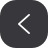被折叠的  条评论 为什么被折叠?到【灌水乐园】发言¥2 ¥4 ¥6 ¥10 ¥20余额支付 (余额：-- )扫码支付获取中扫码支付点击重新获取扫码支付1.余额是钱包充值的虚拟货币，按照1:1的比例进行支付金额的抵扣。
2.余额无法直接购买下载，可以购买VIP、C币套餐、付费专栏及课程。余额充值